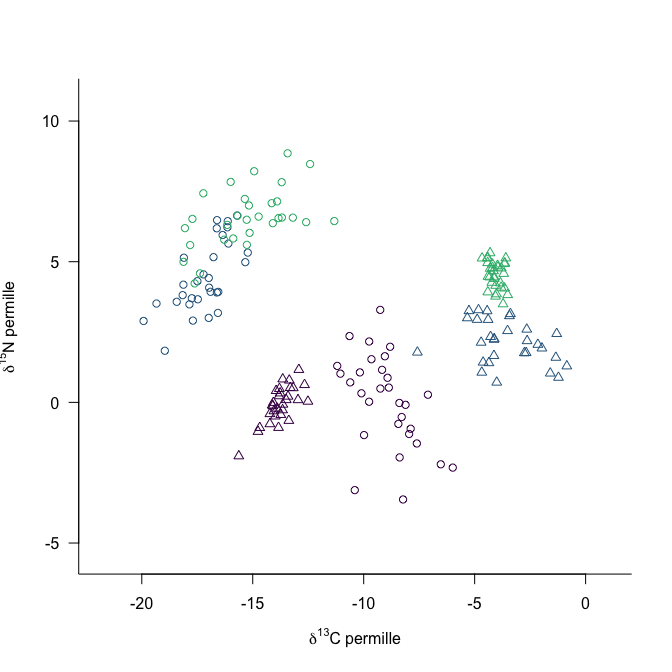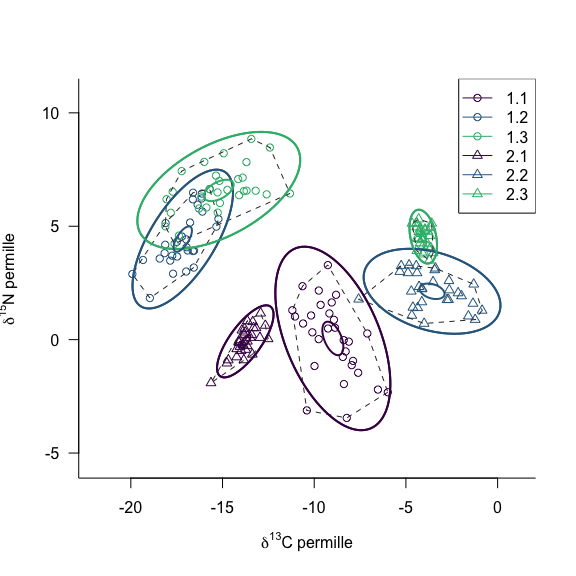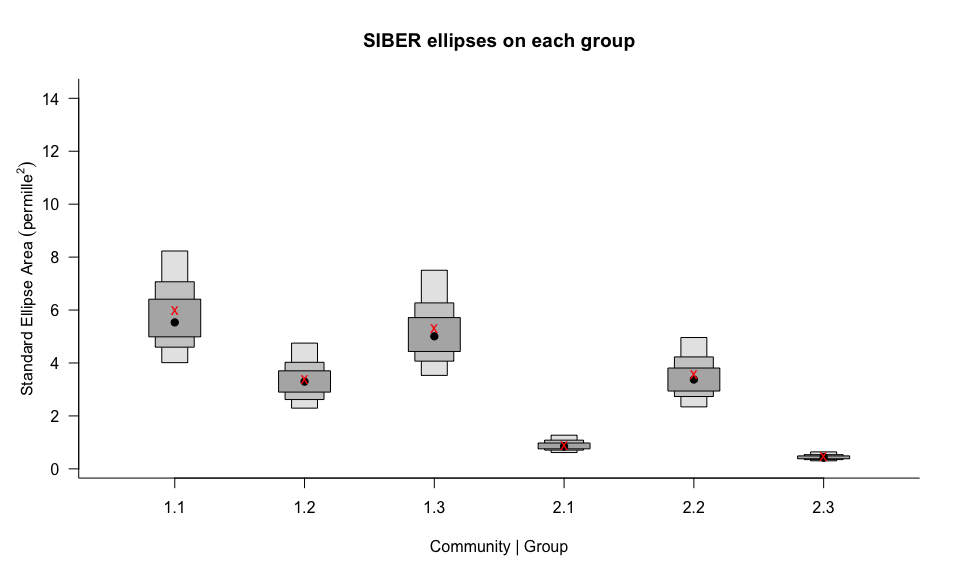# Comparing populations

#### 2023-10-19

Let us return to the original figure we explored in Introduction-to-SIBER where we had multiple populations and we wanted to ask whether they differed in how they are distributed in isotope-space. Specifically we want to test whether two or more ellipses are different from one another.In this figure, we have 4 populations (or more generically: groups) of individuals. We might want to make some comparisons of the isotopic (and hence ecological) niches occupied by these individuals. The two most obvious ways to compare two of these populations, is to ask whether their niches are located in the same place, and if they are the same size. Thereafter, we may be interested in asking to what extent do their niches overlap.

We can visualise this by adding both ellipses, and convex hulls to the data. In this case, we add standard ellipses, which are to bivariate data as standard deviations are to univariate data. A standard ellipse contains approximately 40% of the data, although they can be rescaled to contain any proportion of the data we wish if we accept the assumption that they multivariate normal distributed. Owing to this proportional representation of the data, the ellipse should be insensitive to sample size, and should always contain 40% of the data. However, as was demonstrated in the SIBER paper Jackson et al 2010, the basic Standard Ellipse Area (SEA) shows bias at small sample sizes, which can be corrected to calculate SEAc.

In contrast, the convex hull is a polygon that is drawn around the outermost points in the cloud of data such that all other points lie within the outline. If we were to go out and collect more samples, then this hull can only grow in size and not get smaller. The result of this is that smaller sample sizes will result in smaller convex hulls. Despite this statistical problem, the convex hull remains a useful way to help us visualise bivariate data such as carbon-nitrogen stable isotope values.

We can then go back to our community comprising 4 populations, and add standard ellipses and convex hulls to each group. The new code in SIBER creates a special object that contains the original data, the rescaled and z-scored data, along with various summary statistics to aid with plotting and model fitting. Most of this happens in the background, so you don’t need to worry about the details of this object unless you want to delve deeper.

And now the full code…

rm(list = ls()) # clear the memory of objects

# load the siar package of functions
library(SIBER)

# Replace this line with a call to read.csv() or similar pointing to your
# own dataset.
data("demo.siber.data")
mydata <- demo.siber.data

# create the siber object
siber.example <- createSiberObject(mydata)

# Create lists of plotting arguments to be passed onwards to each
# of the three plotting functions.
community.hulls.args <- list(col = 1, lty = 1, lwd = 1)
group.ellipses.args  <- list(n = 100, p.interval = 0.95,
lty = 1, lwd = 2)
group.hull.args      <- list(lty = 2, col = "grey20")

# ellipses and group.hulls are set to TRUE or T for short to force
# their plotting.
par(mfrow=c(1,1))
plotSiberObject(siber.example,
hulls = F, community.hulls.args,
ellipses = T, group.ellipses.args,
group.hulls = T, group.hull.args,
bty = "L",
iso.order = c(1,2),
xlab = expression({delta}^13*C~'permille'),
ylab = expression({delta}^15*N~'permille')
)

# You can add more ellipses by directly calling plot.group.ellipses()
plotGroupEllipses(siber.example, n = 100, p.interval = 0.95,
lty = 1, lwd = 2)

# or you can add the XX% confidence interval around the bivariate means
# by specifying ci.mean = T along with whatever p.interval you want.
plotGroupEllipses(siber.example, n = 100, p.interval = 0.95,
ci.mean = T, lty = 1, lwd = 2)

# Calculate sumamry statistics for each group: TA, SEA and SEAc
group.ML <- groupMetricsML(siber.example)
print(group.ML)
##            1.1       1.2       1.3       2.1       2.2       2.3
## TA   21.924922 10.917715 17.945127 3.0714363 11.476354 1.4818061
## SEA   5.783417  3.254484  5.131601 0.8623300  3.458824 0.4430053
## SEAc  5.989967  3.370715  5.314872 0.8931275  3.582354 0.4588269
# add a legend
legend("topright", colnames(group.ML),
pch = c(1,1,1,2,2,2), col = c(1:3, 1:3), lty = 1)## Using Bayesian Inference to calculate uncertainty around ellipses

So far these still just point-metrics that describe the width of the isotopic niche. That is, they are single numbers for each group, which means that we can’t compare one group to another in a statistical sense as we lack a measure of the uncertainty around each estimate. This is where we can use Bayesian Inference to quantify the error associated with fitting these ellipses to each group, that arises from both the number of samples we have, and also their distribution.

Essentially, what the MCMC algorithm does is generate a distribution of covariance matrices that to a greater or lesser extent (in terms of likelihood) describe the observed data. It does so, as is the general case in Bayesian Inference, by combing the prior probability with the likelihood of the data for a given covariance matrix.

SIBER uses the jags package to fit the Bayesian model and so we need to specify the parameters of the simulation run, including: run length, burn-in period, number of chains etc…

# options for running jags
parms <- list()
parms$n.iter <- 2 * 10^4 # number of iterations to run the model for parms$n.burnin <- 1 * 10^3 # discard the first set of values
parms$n.thin <- 10 # thin the posterior by this many parms$n.chains <- 2        # run this many chains

# define the priors
priors <- list()
priors$R <- 1 * diag(2) priors$k <- 2
priors$tau.mu <- 1.0E-3 # fit the ellipses which uses an Inverse Wishart prior # on the covariance matrix Sigma, and a vague normal prior on the # means. Fitting is via the JAGS method. ellipses.posterior <- siberMVN(siber.example, parms, priors) ## Compiling model graph ## Resolving undeclared variables ## Allocating nodes ## Graph information: ## Observed stochastic nodes: 30 ## Unobserved stochastic nodes: 3 ## Total graph size: 45 ## ## Initializing model ## ## Compiling model graph ## Resolving undeclared variables ## Allocating nodes ## Graph information: ## Observed stochastic nodes: 30 ## Unobserved stochastic nodes: 3 ## Total graph size: 45 ## ## Initializing model ## ## Compiling model graph ## Resolving undeclared variables ## Allocating nodes ## Graph information: ## Observed stochastic nodes: 30 ## Unobserved stochastic nodes: 3 ## Total graph size: 45 ## ## Initializing model ## ## Compiling model graph ## Resolving undeclared variables ## Allocating nodes ## Graph information: ## Observed stochastic nodes: 30 ## Unobserved stochastic nodes: 3 ## Total graph size: 45 ## ## Initializing model ## ## Compiling model graph ## Resolving undeclared variables ## Allocating nodes ## Graph information: ## Observed stochastic nodes: 30 ## Unobserved stochastic nodes: 3 ## Total graph size: 45 ## ## Initializing model ## ## Compiling model graph ## Resolving undeclared variables ## Allocating nodes ## Graph information: ## Observed stochastic nodes: 30 ## Unobserved stochastic nodes: 3 ## Total graph size: 45 ## ## Initializing model What we end up with is a range of ellipses that could explain the data, with more of them clustered around the most likely solution. However, one cannot simply take an average across these covariance matrices, as there are strict mathematical properties that must be maintained. The result of this is that it is not possible to plot a mean, median or modal Bayesian Standard Ellipse; instead we must calculate each one of the ellipse’s area, and then present summary statistics of this derived measurement. SIBER contains a function that will automatically loop over all the groups and do this. The plots below represent the posterior distribution of the SEA_B fitted to each of the 4 groups in our dataset. # # ---------------------------------------------------------------- # Plot out some of the data and results # ---------------------------------------------------------------- # The posterior estimates of the ellipses for each group can be used to # calculate the SEA.B for each group. SEA.B <- siberEllipses(ellipses.posterior) siberDensityPlot(SEA.B, xticklabels = colnames(group.ML), xlab = c("Community | Group"), ylab = expression("Standard Ellipse Area " ('permille' ^2) ), bty = "L", las = 1, main = "SIBER ellipses on each group" ) # Add red x's for the ML estimated SEA-c points(1:ncol(SEA.B), group.ML[3,], col="red", pch = "x", lwd = 2)# Calculate some credible intervals cr.p <- c(0.95, 0.99) # vector of quantiles # call to hdrcde:hdr using lapply() SEA.B.credibles <- lapply( as.data.frame(SEA.B), function(x,...){tmp<-hdrcde::hdr(x)$hdr},
prob = cr.p)

print(SEA.B.credibles)
## $V1 ## [,1] [,2] ## 99% 3.407949 9.338847 ## 95% 3.964803 8.212741 ## 50% 5.005908 6.433000 ## ##$V2
##         [,1]     [,2]
## 99% 1.968820 5.309634
## 95% 2.236672 4.709673
## 50% 2.904874 3.713784
##
## $V3 ## [,1] [,2] ## 99% 3.028268 8.332197 ## 95% 3.476047 7.435144 ## 50% 4.454608 5.734916 ## ##$V4
##          [,1]      [,2]
## 99% 0.5478255 1.4430533
## 95% 0.6105722 1.2655676
## 50% 0.7600099 0.9806027
##
## $V5 ## [,1] [,2] ## 99% 2.094540 5.517873 ## 95% 2.322945 4.944584 ## 50% 2.964104 3.834244 ## ##$V6
##          [,1]      [,2]
## 99% 0.2681770 0.7254754
## 95% 0.3003982 0.6384048
## 50% 0.3815994 0.4913769
# do similar to get the modes, taking care to pick up multimodal posterior
# distributions if present
SEA.B.modes <- lapply(
as.data.frame(SEA.B),
function(x,...){tmp<-hdrcde::hdr(x)$mode}, prob = cr.p, all.modes=T) print(SEA.B.modes) ##$V1
##  5.653001
##
## $V2 ##  3.30809 ## ##$V3
##  5.050318
##
## $V4 ##  0.8572488 ## ##$V5
##  3.368257
##
## \$V6
##  0.4325926

## Comparing the posterior distributions

In order to test whether one group’s ellipse is smaller or larger than another, we can simply calculate the probability that its posterior distribution is smaller (or larger). This is achieved by comparing each pair of posterior draws for both groups, and determining which is smaller in magnitude. We then find the proportion of draws that are smaller, and this is a direct proxy for the probability that one group’s posterior distribution (of ellipse size in this case) is smaller than the other.

Here, we first calculate the proportion, and hence probability, of the SEA.B for group 1 being smaller than the SEA.B for group 2.

Pg1.1_lt_g1.2 <- sum( SEA.B[,1] < SEA.B[,2] ) / nrow(SEA.B)
print(Pg1.1_lt_g1.2)
##  0.018

So, in this case, less than 2% of groups 1’s ellipse are smaller than for group 2, which implies that 98% of the posterior ellipses are larger for group 2.1; which matches well with what we can see in the density plot above where there is little overlap between the 95% credible intervals.

Then we can do exactly the same for groups 1.1 and 1.3.

Pg1.1_lt_g1.3 <- sum( SEA.B[,1] < SEA.B[,3] ) / nrow(SEA.B)
print(Pg1.1_lt_g1.3)
##  0.32725

And then for some of the other pairings:

Pg1.1_lt_g2.1 <- sum( SEA.B[,1] < SEA.B[,4] ) / nrow(SEA.B)
print(Pg1.1_lt_g2.1)
##  0
Pg1.2_lt_g1.3 <- sum( SEA.B[,2] < SEA.B[,3] ) / nrow(SEA.B)
print(Pg1.2_lt_g1.3)
##  0.95525
Pg1.3_lt_g2.1 <- sum( SEA.B[,3] < SEA.B[,4] ) / nrow(SEA.B)
print(Pg1.3_lt_g2.1)
##  0
Pg2.2_lt_g2.3 <- sum( SEA.B[,5] < SEA.B[,6] ) / nrow(SEA.B)
print(Pg2.2_lt_g2.3)
##  0

## Overlap Between Ellipses

One can calculate the overlap between two (or more) ellipses. In the first instance, this overlap is simply the area, in units of per mil squared, contained by the shape that lies within the overlapping region. This overlap is most easily calculated by using the SEAc of each ellipse.

The overlap between the SEAc for groups 1.2 and 1.3 is given by:

overlap.G1.2.G1.3 <- maxLikOverlap("1.2", "1.3", siber.example, p = 0.95, n =)

One might then wish to calculate the proportion overlap; although one then runs into a choice as to what the denominator will be in the equation. You could for instance calculate the proportion of A that overlaps with B, the proportion of B that overlaps with A, or the proportion of A and B that overlap with each other.

prop.of.first <- as.numeric(overlap.G1.2.G1.3["overlap"] / overlap.G1.2.G1.3["area.1"])
print(prop.of.first)
##  0.5716275
prop.of.second <- as.numeric(overlap.G1.2.G1.3["overlap"] / overlap.G1.2.G1.3["area.2"])
print(prop.of.second)
##  0.3625287
prop.of.both <- as.numeric(overlap.G1.2.G1.3["overlap"] / (overlap.G1.2.G1.3["area.1"] + overlap.G1.2.G1.3["area.2"]))
print(prop.of.both)
##  0.221838

A problem with this simple overlap calculation is that it yields a point-estimate of overlap based on the maximum likelihood estimated SEA_c. One can instead calculate a distribution of overlap based on the posterior distributions of the fitted ellipses. It can be a bit slow to calculate this overlap, so you may want to drop the number of draws if your computer is slow.

bayes.overlap.G2.G3 <- bayesianOverlap("1.2", "1.3", ellipses.posterior,
draws = 10, p.interval = 0.95,
n = 360)
print(bayes.overlap.G2.G3)
##       area1    area2   overlap
## 1  19.19851 34.75983 11.588262
## 2  18.09400 33.30468 10.041812
## 3  22.86408 30.16677 13.692733
## 4  21.08490 31.74608 11.182490
## 5  23.31088 31.50132 13.515079
## 6  11.80408 31.24096  6.374521
## 7  21.85761 40.61769 12.243514
## 8  16.94563 39.97842 11.006042
## 9  24.34104 23.89482 10.746677
## 10 12.05999 21.61679  6.618486